# 连续Sylvester矩阵方程求解的分裂迭代算法*

(商丘师范学院 信息技术学院， 河南 商丘 476000)

AX+XB=F

(1)

## 1 分裂迭代算法

### 1.1 外迭代格式

A=M1-N1B=M2-N2

(2)

M1=(AT+A)/2， N1=(AT-A)/2， M2=(BT+B)/2， N2=(BT-B)/2，

(3)

M1X+XM2=N1X+XN2+F.

(4)

M1Xk+1+Xk+1M2=N1Xk+XkN2+F.

(5)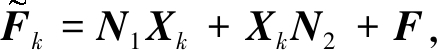则有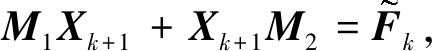(6)

### 1.2 内迭代格式

1.2.1 复对称线性变换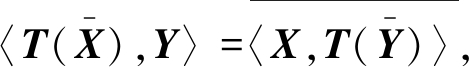(7)

T(X)=C,

(8)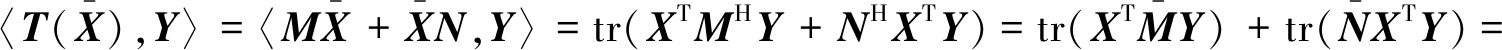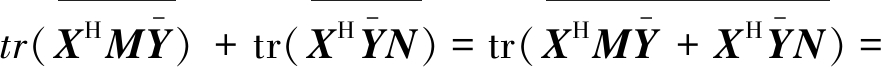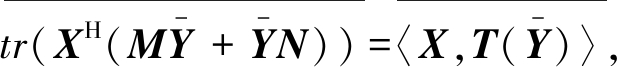1.2.2 算法延拓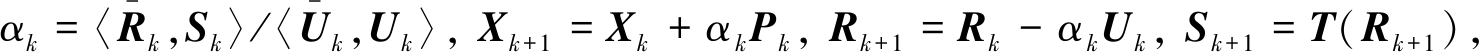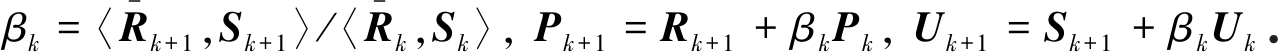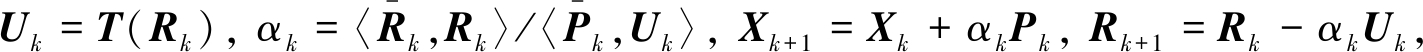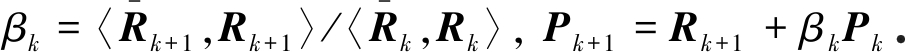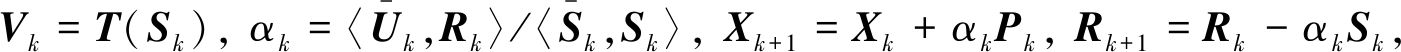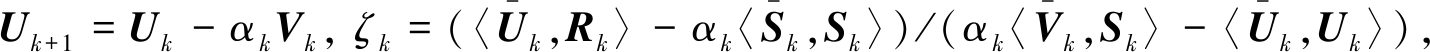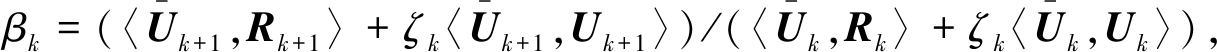Pk+1=Rk+1+βkPkSk+1=Uk+1+βkSk.

### 1.3 分裂迭代算法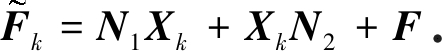## 2 收敛性分析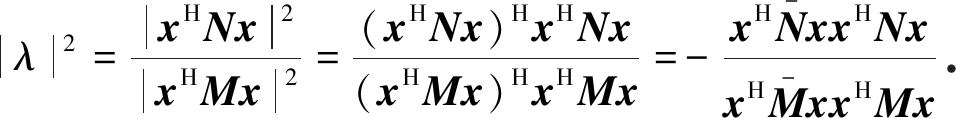(9)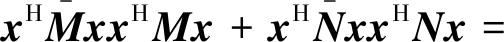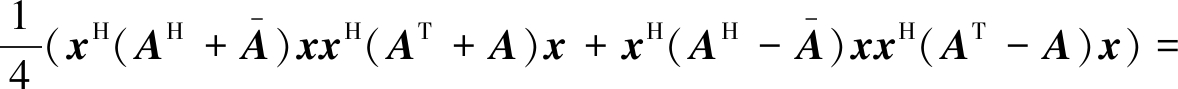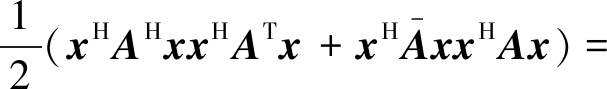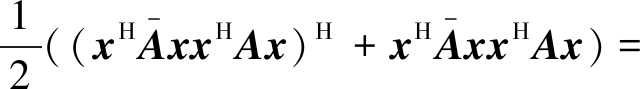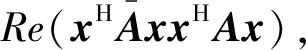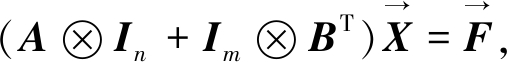(10)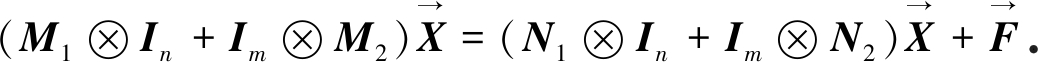(11)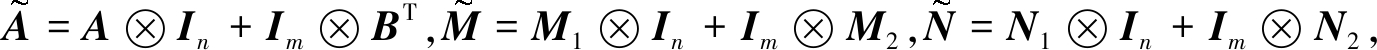则满足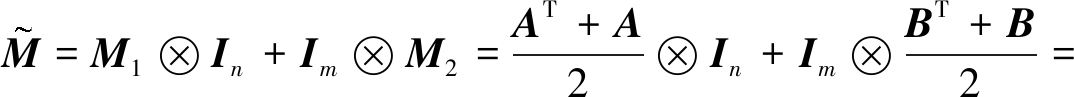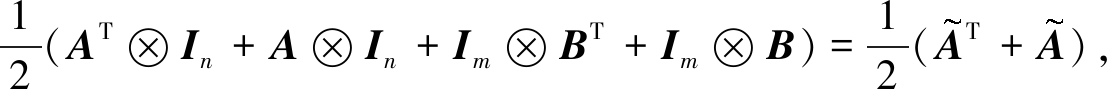(12)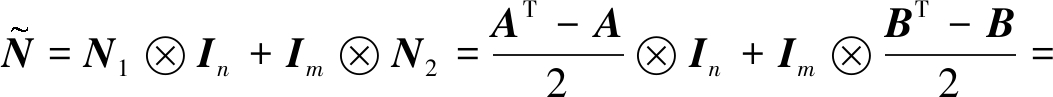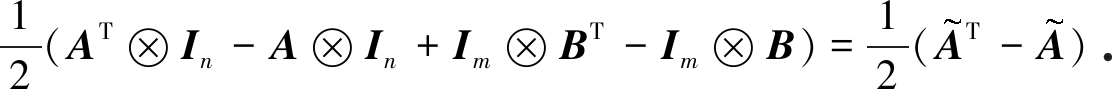(13)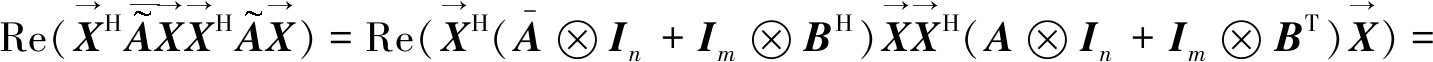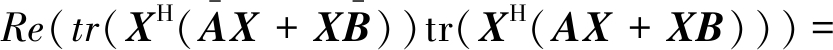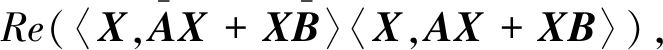(14)

ρ((M1In+ImM2)-1(N1In+ImN2))<1.

## 3 数值算例与结果分析

A=MA+2qNA+100I/(n+1)2B=MB+2qNB+100I/(n+1)2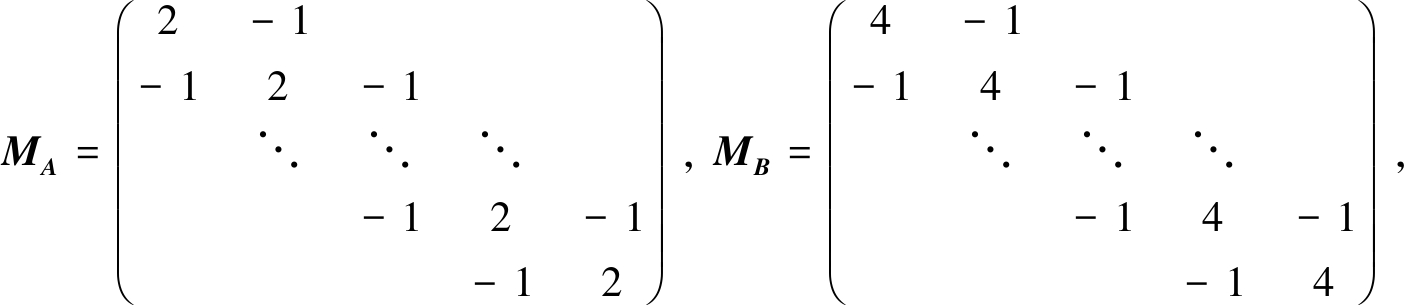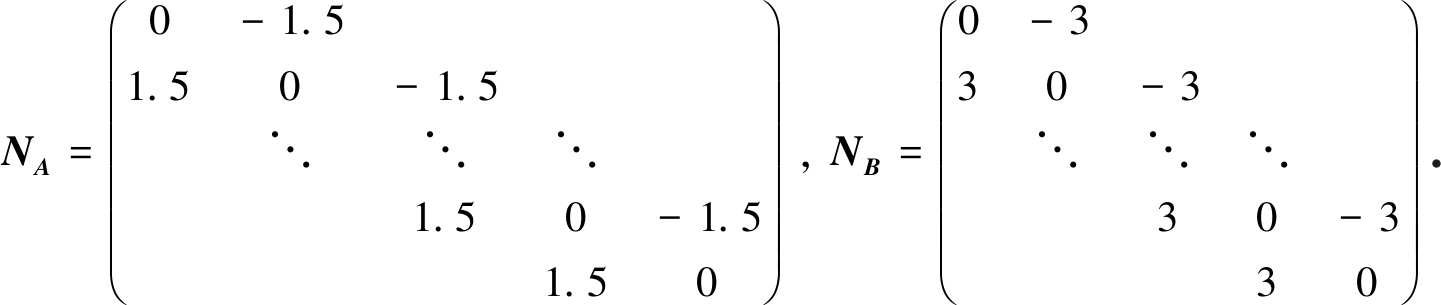Table 1 The theoretical quasi-optimal iteration parameters for INPHSS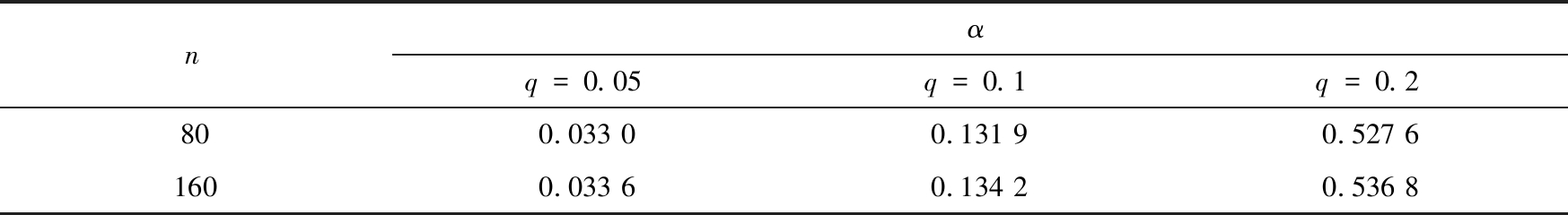nαq=0.05q=0.1q=0.2800.033 00.131 90.527 61600.033 60.134 20.536 8

Table 2 Numerical results of example 1 obtained with the INPHSS，CGS，TS_COCR，TS_COCG

and TS_CCC_SCBiCR methods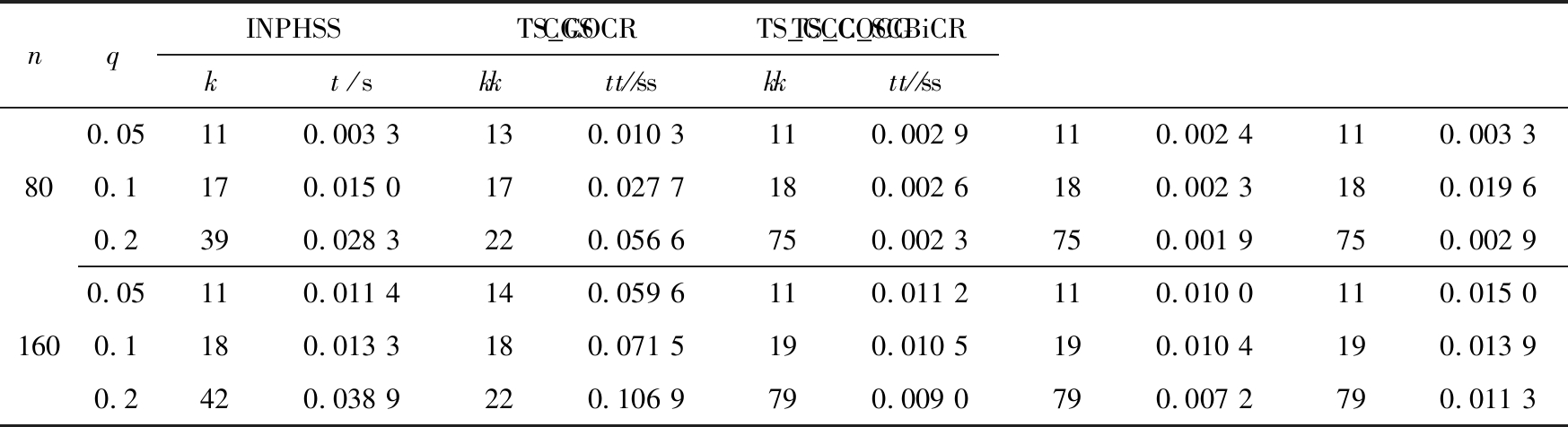nqINPHSSkt/sCGSkt/sTS_COCRkt/sTS_COCGkt/sTS_CCC_SCBiCRkt/s800.05110.003 3130.010 3110.002 9110.002 4110.003 30.1170.015 0170.027 7180.002 6180.002 3180.019 60.2390.028 3220.056 6750.002 3750.001 9750.002 91600.05110.011 4140.059 6110.011 2110.010 0110.015 00.1180.013 3180.071 5190.010 5190.010 4190.013 90.2420.038 9220.106 9790.009 0790.007 2790.011 3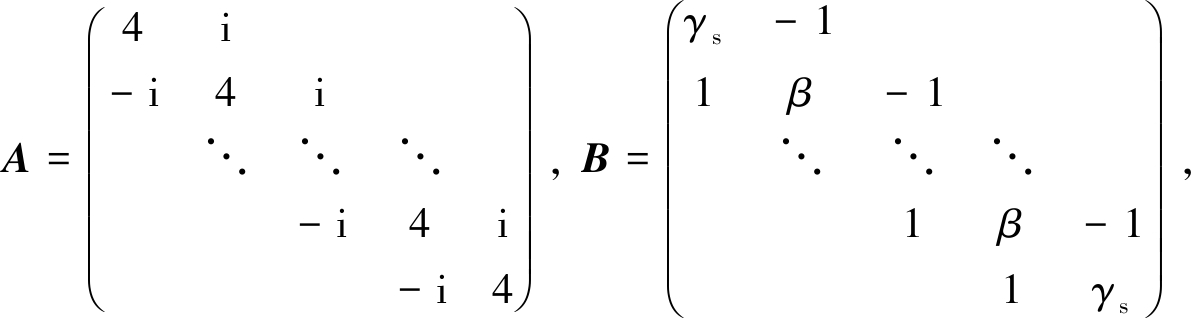Table 3 Numerical results of example 2 obtained with the CGS，TS_COCR，TS_COCG

and TS_CCC_SCBiCR methods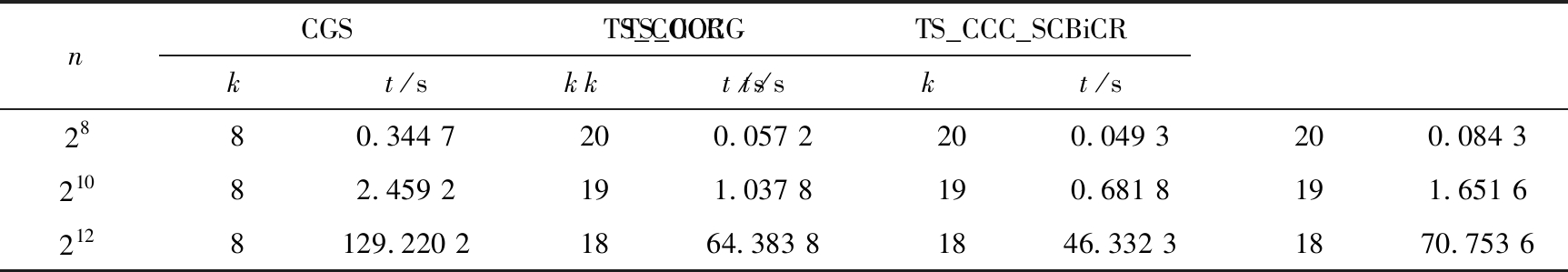nCGSkt/sTS_COCRkt/sTS_COCGkt/sTS_CCC_SCBiCRkt/s2880.344 7200.057 2200.049 3200.084 321082.459 2191.037 8190.681 8191.651 62128129.220 21864.383 81846.332 31870.753 6

A=triu(rand(n),1)×i+diag(qIn+diag(rand(n)))，

B=tril(rand(n),1)+diag(qIn+diag(rand(n)))×5i，

1) 表1表明随着参数q的增加，系数矩阵AB次对角线元素增大，进而INPHSS算法所涉及的理论拟最优参数值增加，且在同一矩阵维数下，INPHSS算法的迭代次数和迭代时间也随着参数q的增加而增加；相较于CGS算法和TS_CCC_SCBiCR算法，INPHSS算法具有良好的收敛性，然而TS_COCG算法和TS_COCR算法的收敛速度明显优于INPHSS算法，并且避免了最优迭代参数的选取.

2) 表2～4的数值结果表明，相较于直接求解矩阵方程(1)的CGS算法，分裂迭代法(TS_COCR、TS_COCG、TS_CCC_SCBiCR)明显提高了方程(1)的求解速度；此外通过观察文中所提出的分裂迭代法，可以发现随着阶数n的增加，TS_COCG算法具有良好的收敛性，特别是例2，当矩阵阶数达到212时，TS_COCG算法相较于TS_COCR和TS_CCC_SCBiCR算法，迭代速度加快了至少0.25倍，这也进一步说明了TS_COCG算法的有效性和优越性.

Table 4 Numerical results of example 3 obtained with the CGS，TS_COCR，TS_COCG

and TS_CCC_SCBiCR methodsnCGSkt/sTS_COCRkt/sTS_COCGkt/sTS_CCC_SCBiCRkt/s10070.014 5180.007 6180.006 6180.012 120080.056 3240.046 9240.039 9240.063 740090.337 0390.335 5390.308 7390.438 2

## 4 结 束 语

1) 相较于传统的CGS算法，分裂迭代算法(TS_COCR、TS_COCG、TS_CCC_SCBiCR)因其利用了系数矩阵分裂后复对称的特点，具有良好的收敛性；此外，所提出的算法与传统的分裂思想不同(分裂为Hermite矩阵和反Hermite矩阵)，巧妙避免了最优迭代参数的选取，提高了算法的易实现性、易操作性.

2) 分裂迭代算法由内外迭代格式组成，并且整体算法的收敛性很大程度依赖于内迭代格式的选取，而COCG法和COCR法相较于CCC_SCBiCR法具有较好的收敛性和鲁棒性，因此，从理论层面来看TS_COCR和TS_COCG算法的收敛速度优于TS_CCC_SCBiCR算法的收敛速度，同时数值算例也验证了这一点.

 LANCASTER P, RODMAN L. Algebraic Riccati Equations[M]. Oxford: The Clarendon Press, 1995.

 CHIANG C Y. On the Sylvester-like matrix equation AX+f(X)B=C[J]. Journal of the Franklin Institute, 2016, 353: 1061-1074.

 CALVETTI D, REICHEL L. Application of ADI iterative methods to the restoration of noisy images[J]. SIAM Journal on Matrix Analysis and Applications, 1996, 17(1): 165-186.

 ANDERSON B, AGATHOKLIS P, JURY E, et al. Stability and the matrix Lyapunov equation for discrete 2-dimensional systems[J]. IEEE Transactions on Circuits and Systems, 1986, 33(3): 261-267.

 BARTELS R H, STEWART G W. Solution of the matrix equation AX+XB=C[F4][J]. Communications of the ACM, 1972, 15(9): 820-826.

 GOLUB G, NASH S, VAN LOAN C. A Hessenberg-Schur method for the problem AX+XB=C[J]. IEEE Transactions on Automatic Control, 1979, 24(6): 909-913.

 BAI Z Z. On Hermitian and skew-Hermitian splitting iteration methods for continuous Sylvester equations[J]. Journal of Computational Mathematics, 2011, 29(2): 185-198.

 ZHOU D M, CHEN G L, CAI Q Y. On modified HSS iteration methods for continuous Sylvester equations[J]. Applied Mathematics and Computation, 2015, 263: 84-93.

 XU L, HUO H F, YANG A L. Preconditioned HSS iteration method and its non-alternating variant for continuous Sylvester equations[J]. Computers and Mathematics With Applications, 2018, 75(4): 1095-1106.

 DEHGHAN M, SHIRILORD A. A generalized modified Hermitian and skew-Hermitian splitting (GMHSS) method for solving complex Sylvester matrix equation[J]. Applied Mathematics and Computation, 2019, 348: 632-651.

 ZHOU R, WANG X, TANG X B. A generalization of the Hermitian and skew-Hermitian splitting iteration method for solving Sylvester equations[J]. Applied Mathematics and Computation, 2015, 271: 609-617.

 ZHOU R, WANG X, TANG X B. Preconditioned positive-definite and skew-Hermitian splitting iteration methods for continuous Sylvester equations AX+XB=C[J]. East Asian Journal on Applied Mathematics, 2017, 7(1): 55-69.

 ZHENG Q Q, MA C F. On normal and skew-Hermitian splitting iteration methods for large sparse continuous Sylvester equations[J]. Journal of Computational and Applied Mathematics, 2014, 268: 145-154.

 XIAO X Y, WANG X, YIN H W. Efficient single-step preconditioned HSS iteration methods for complex symmetric linear systems[J]. Computers and Mathematics With Applications, 2017, 74(10): 2269-2280.

 XIAO X Y, WANG X, YIN H W. Efficient preconditioned NHSS iteration methods for solving complex symmetric linear systems[J]. Computers and Mathematics With Applications, 2018, 75(1): 235-247.

 杨丽, 李军. Hilbert空间中分裂可行性问题的改进Halpern迭代和黏性逼近算法[J]. 应用数学和力学, 2017, 38(9): 1072-1080.(YANG Li, LI Jun. Modified Halpern iteration and viscosity approximation methods for split feasibility problems in Hilbert spaces[J]. Applied Mathematics and Mechanics, 2017, 38(9): 1072-1080.(in Chinese))

 LI C L, MA C F. On semi-convergence of parameterized SHSS method for a class of singular complex symmetric linear systems[J]. Computers and Mathematics With Applications, 2019, 77: 466-475.

 HUANG Z G, WANG L G, XU Z, et al. The generalized double steps scale-SOR iteration method for solving complex symmetric linear systems[J]. Journal of Computational and Applied Mathematics, 2019, 346: 284-306.

 HUANG Z G, WANG L G, XU Z, et al. Preconditioned accelerated generalized successive overrelaxation method for solving complex symmetric linear systems[J]. Computers and Mathematics With Applications, 2019, 77(7): 1902-1916.

 VAN DER VORST H A, MELISSEN J B M. A Petrov-Galerkin type method for solving Ax=b, where A is symmetric complex[J]. IEEE Transactions on Magnetics, 1990, 26(2): 706-708.

 GU X M, CLEMENS M, HUANG T Z, et al. The SCBiCG class of algorithms for complex symmetric linear systems with applications in several electromagnetic model problems[J]. Computer Physics Communications, 2015, 191: 52-64.

 SOGABE T, ZHANG S L. A COCR method for solving complex symmetric linear systems[J]. Journal of Computational and Applied Mathematics, 2007, 199(2): 297-303.

 CLEMENS M, WEILAND T, VAN RIENEN U. Comparison of Krylov-type methods for complex linear systems applied to high-voltage problems[J]. IEEE Transactions on Magnetics, 1998, 34(5): 3335-3338.

 ABE K, FUJINO S. Converting BiCR method for linear equations with complex symmetric matrices[J]. Applied Mathematics and Computation, 2018, 321: 564-576.

 HAJARIAN M. Matrix form of the CGS method for solving general coupled matrix equations[J]. Applied Mathematics Letters, 2014, 34: 37-42.

# A Splitting Iterative Algorithm for Solving Continuous Sylvester Matrix Equations

LI Ying

(School of Information Technology, Shangqiu Normal University, Shangqiu, Henan 476000, P.R.China)

Abstract: The solution of continuous Sylvester matrix equations has significant application value in scientific and engineering calculations, hence, a splitting iterative algorithm was proposed. The core idea of the algorithm is to split the coefficient matrix of the continuous Sylvester matrix equation into a symmetric matrix and an antisymmetric matrix with an outer iterative scheme, and to solve the complex symmetric matrix equation with the inner iterative scheme. Compared with the traditional splitting algorithms, the proposed splitting algorithm effectively avoids the selection of optimal iterative parameters and takes advantages of the efficient solution of complex symmetric equations, which improves the easy implementation and easy operation of the algorithm. In addition, the convergence of the splitting iterative algorithm was further proved theoretically. Numerical examples show that, the splitting iterative algorithm has good convergence and robustness, and the convergence of the splitting iterative algorithm depends on the selection of the inner iterative schemes.

Key words: Sylvester matrix equation; complex symmetric matrix equation; splitting iterative algorithm; convergence

ⓒ 应用数学和力学编委会，ISSN 1000-0887

http://www.applmathmech.cn

*收稿日期： 2019-04-04；

DOI: 10.21656/1000-0887.400133